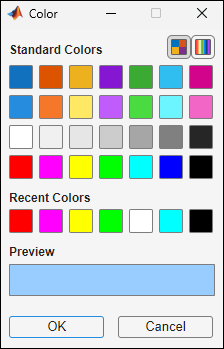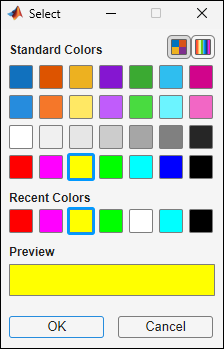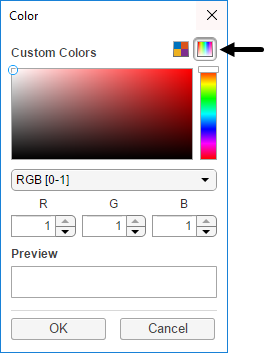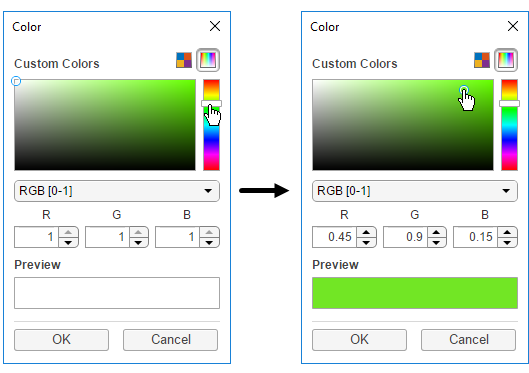# uisetcolor

## 语法

``c = uisetcolor``
``c = uisetcolor(RGB)``
``c = uisetcolor(obj)``
``c = uisetcolor(___,title)``

## 说明

``c = uisetcolor` 显示模态颜色选择器，并以 RGB 三元组形式返回所选颜色。RGB 三元组是三元素行向量，其元素指定颜色的红、绿和蓝分量的强度。这些强度处于范围 [0,1] 中，类型为 `double`。`

``c = uisetcolor(RGB)` 指定 RGB 三元组形式的默认颜色选择。`
``c = uisetcolor(obj)` 将默认颜色选择设置为某个对象（例如 `Figure`）的颜色。如果从颜色选择器中选择不同的颜色，点击确定后，对象的颜色将更改为新颜色。对象必须有一个属性控制颜色的某些方面。例如，某些对象具有 `Color` 或 `BackgroundColor` 属性。`

``c = uisetcolor(___,title)` 为对话框指定自定义标题。将标题指定为上述任一语法的最后一个参数。`

## 示例

`c = uisetcolor([0.6 0.8 1])````c = 0.6000 0.8000 1.0000```

`c = uisetcolor([1 1 0],'Select a color')````c = 1 1 0```

`c = uisetcolor````c = 0.4471 0.9020 0.1451```